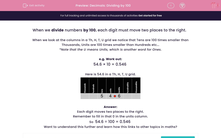# Decimals: Dividing by 100

In this worksheet, stidemts divide numbers by 100 by shifting digits two places to the right in a grid.Key stage:  KS 2

Curriculum topic:   Maths and Numerical Reasoning

Curriculum subtopic:   Decimals

Difficulty level:#### Worksheet Overview

When we divide numbers by 100, each digit must move two places to the right.

When we look at the columns in a Th, H, T, U grid we notice that Tens are 100 times smaller than Thousands, Units are 100 times smaller than Hundreds etc...

*Note that the U means Units, which is another word for Ones.

e.g. Work out:

54.6 × 10 = 0.546

Here is 54.6 in a Th, H, T, U grid.Each digit moves two places to the right.

Remember to fill in that 0 in the units column.

So  54.6 ÷ 100 = 0.546

Want to understand this further and learn how this links to other topics in maths?

### What is EdPlace?

We're your National Curriculum aligned online education content provider helping each child succeed in English, maths and science from year 1 to GCSE. With an EdPlace account you’ll be able to track and measure progress, helping each child achieve their best. We build confidence and attainment by personalising each child’s learning at a level that suits them.

Get started